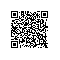# C语言例题25：

题目要求：一维数组实现杨辉三角

#include <stdio.h> void main() { int i,j,x; //x,y是二个计数器，X是欲显示的行数 scanf("%d",&x); int a={1}; int b={1}; for(i=0;i<x;i++) { for(j=0;j<=i;j++) { printf("%4d ",a[j]); //A数组是真正显示的数组 b[j+1]=a[j]+a[j+1]; //B数组是通过A数组计算出来的，其实也就是要显示的下一行的内容 a[j]=b[j]; //将数组B的内容重新拷回到数组A中，因为我们要显示的是A，而不是B } a[j]=1; //数组末尾封1结束 printf("/n"); //换行 } }使用钉钉扫一扫加入圈子
+ 订阅